## Distance, Velocity, and Acceleration

The indefinite integral is commonly applied in problems involving distance, velocity, and acceleration, each of which is a function of time. In the discussion of the applications of the derivative, note that the derivative of a distance function represents instantaneous velocity and that the derivative of the velocity function represents instantaneous acceleration at a particular time. In considering the relationship between the derivative and the indefinite integral as inverse operations, note that the indefinite integral of the acceleration function represents the velocity function and that the indefinite integral of the velocity represents the distance function.

In case of a free‐falling object, the acceleration due to gravity is –32 ft/sec 2. The significance of the negative is that the rate of change of the velocity with respect to time (acceleration), is negative because the velocity is decreasing as the time increases. Using the fact that the velocity is the indefinite integral of the acceleration, you find thatNow, at t = 0, the initial velocity ( v 0) ishence, because the constant of integration for the velocity in this situation is equal to the initial velocity, write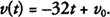Because the distance is the indefinite integral of the velocity, you find thatNow, at t = 0, the initial distance ( s 0) is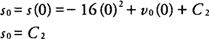hence, because the constant of integration for the distance in this situation is equal to the initial distance, writeExample 1: A ball is thrown downward from a height of 512 feet with a velocity of 64 feet per second. How long will it take for the ball to reach the ground?

From the given conditions, you find that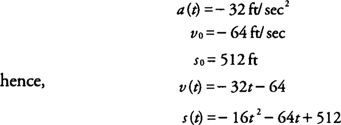The distance is zero when the ball reaches the ground orhence, the ball will reach the ground 4 seconds after it is thrown.

Example 2: In the previous example, what will the velocity of the ball be when it hits the ground?

Because v( t) = –32( t) – 64 and it takes 4 seconds for the ball to reach the ground, you find that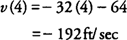hence, the ball will hit the ground with a velocity of –192 ft/sec. The significance of the negative velocity is that the rate of change of the distance with respect to time (velocity) is negative because the distance is decreasing as the time increases.

Example 3: A missile is accelerating at a rate of 4 t m/sec 2 from a position at rest in a silo 35 m below ground level. How high above the ground will it be after 6 seconds?

From the given conditions, you find that a( t) = 4 t m/sec 2, v 0 = 0 m/sec because it begins at rest, and s 0 = –35 m because the missile is below ground level; hence,After 6 seconds, you find that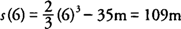hence, the missile will be 109 m above the ground after 6 seconds.

Back to Top
A18ACD436D5A3997E3DA2573E3FD792A